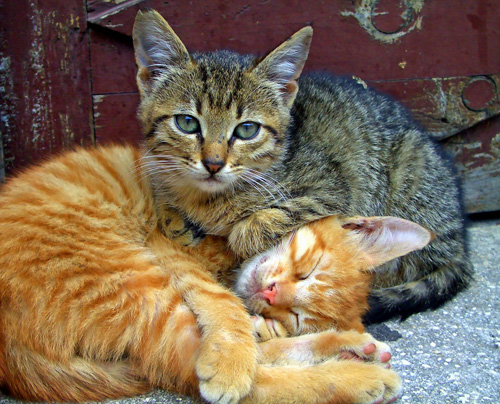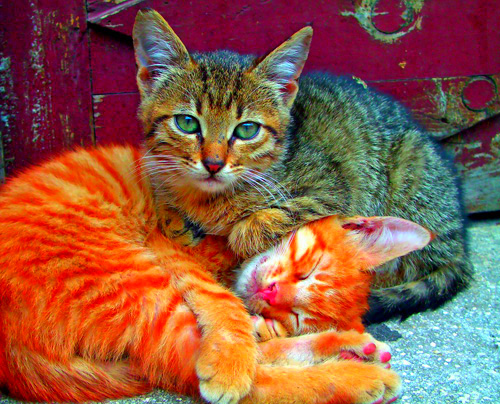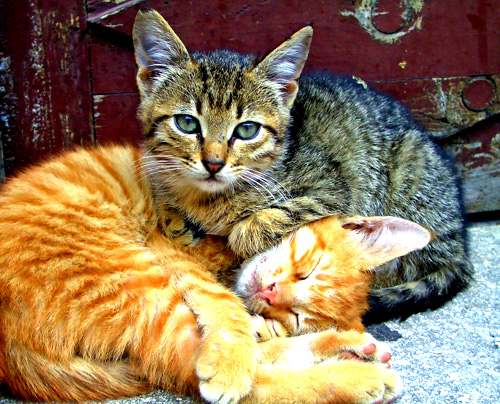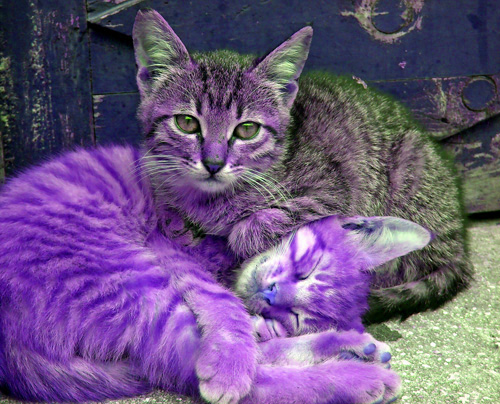# Understanding AS3 ColorMatrixFilter class

Do you want to apply color correction to images into your Flash movies on the fly?

Then `ColorMatrixFilter` is the class you need.

The ColorMatrixFilter class lets you apply a 4 x 5 matrix transformation on the RGBA color and alpha values of every pixel in the input image to produce a result with a new set of RGBA color and alpha values.

The color matrix filter separates each source pixel into its red, green, blue, and alpha components as srcR, srcG, srcB, srcA.

To calculate the result of each of the four channels, the value of each pixel in the image is multiplied by the values in the transformation matrix.

It allows saturation changes, hue rotation, luminance to alpha, and various other effects.

Around the web you can find a lot of examples of photos loaded with Flash and sliders to change colors, but I want to explore this feature from a coding point of view.

I will make some experiment on this photo I found on FlickrThe first thing is creating a MovieClip with the image inside. I called it `cats`, so with this simple code I display the cats on the stage:

```package {
import flash.display.Sprite;
public class cmf extends Sprite {
var cats_image:cats=new cats();
public function cmf() {
}
}
}
```

nothing new… now it’s time to add the filter.

Now let’s try this script:

```package {
import flash.display.Sprite;
import flash.filters.ColorMatrixFilter;
public class cmf extends Sprite {
var cats_image:cats=new cats();
public function cmf() {
var matrix:Array = new Array();
matrix=matrix.concat([0.5,0.5,0.5,0,0]);// red
matrix=matrix.concat([0.5,0.5,0.5,0,0]);// green
matrix=matrix.concat([0.5,0.5,0.5,0,0]);// blue
matrix=matrix.concat([0,0,0,1,0]);// alpha
var my_filter:ColorMatrixFilter=new ColorMatrixFilter(matrix);
cats_image.filters=[my_filter];
}
}
}
```

multiplying all values by 0.5, we have a standard (and not so interesting) black and white photo.While with this script

```package {
import flash.display.Sprite;
import flash.filters.ColorMatrixFilter;
public class cmf extends Sprite {
var cats_image:cats=new cats();
public function cmf() {
var matrix:Array = new Array();
matrix=matrix.concat([1,0,0,0,0]);// red
matrix=matrix.concat([0,1,0,0,0]);// green
matrix=matrix.concat([0,0,1,0,0]);// blue
matrix=matrix.concat([0,0,0,1,0]);// alpha
var my_filter:ColorMatrixFilter=new ColorMatrixFilter(matrix);
cats_image.filters=[my_filter];
}
}
}
```

we have no changes. Why not? because the matrix is an identity matrix.

An identity matrix or unit matrix of size n is the n-by-n square matrix with ones on the main diagonal and zeros elsewhere.

Changing the saturation

To change the saturation of an image, simply boost red component on red channel, green component on green channel and blue component on blue channel, while decreasing the other ones.

```package {
import flash.display.Sprite;
import flash.filters.ColorMatrixFilter;
public class cmf extends Sprite {
var cats_image:cats=new cats();
public function cmf() {
var matrix:Array = new Array();
matrix=matrix.concat([2,-1,0,0,0]);// red
matrix=matrix.concat([-1,2,0,0,0]);// green
matrix=matrix.concat([0,-1,2,0,0]);// blue
matrix=matrix.concat([0,0,0,1,0]);// alpha
var my_filter:ColorMatrixFilter=new ColorMatrixFilter(matrix);
cats_image.filters=[my_filter];
}
}
}
```To add contrast, increase red component on red channel, green component on green channel and blue component on blue channel and decrease all offset values, this way

```package {
import flash.display.Sprite;
import flash.filters.ColorMatrixFilter;
public class cmf extends Sprite {
var cats_image:cats=new cats();
public function cmf() {
var matrix:Array = new Array();
matrix=matrix.concat([1.5,0,0,0,-40]);// red
matrix=matrix.concat([0,1.5,0,0,-40]);// green
matrix=matrix.concat([0,0,1.5,0,-40]);// blue
matrix=matrix.concat([0,0,0,1,0]);// alpha
var my_filter:ColorMatrixFilter=new ColorMatrixFilter(matrix);
cats_image.filters=[my_filter];
}
}
}
```Changing the hue

To change the hue, swap red, green and blue values

```package {
import flash.display.Sprite;
import flash.filters.ColorMatrixFilter;
public class cmf extends Sprite {
var cats_image:cats=new cats();
public function cmf() {
var matrix:Array = new Array();
matrix=matrix.concat([0,1,0,0,0]);// red
matrix=matrix.concat([0,0,1,0,0]);// green
matrix=matrix.concat([1,0,0,0,0]);// blue
matrix=matrix.concat([0,0,0,1,0]);// alpha
var my_filter:ColorMatrixFilter=new ColorMatrixFilter(matrix);
cats_image.filters=[my_filter];
}
}
}
```Obviously you must finetune your matrix in order to achieve the best effect, but now you know how to change colors on the fly.

215 GAME PROTOTYPES EXPLAINED WITH SOURCE CODE
// 1+2=3
// 10000000
// 2 Cars
// 2048
// Avoider
// Ballz
// Block it
// Blockage
// Bloons
// Boids
// Bombuzal
// Breakout
// Bricks
// Columns
// CubesOut
// Dots
// DROP'd
// Dudeski
// Eskiv
// Filler
// Fling
// Globe
// HookPod
// Hundreds
// InkTd
// Iromeku
// Lumines
// Magick
// MagOrMin
// Maze
// Memdot
// Nano War
// Nodes
// o:anquan
// Ononmin
// Pacco
// Phyballs
// Platform
// Poker
// Pool
// Poux
// Pudi
// qomp
// Racing
// Renju
// SameGame
// Security
// Sling
// Slingy
// Sokoban
// Splitter
// Sproing
// Stack
// Stairs
// Stringy
// Sudoku
// Tetris
// Threes
// Toony
// Turn
// TwinSpin
// vvvvvv
// Wordle
// Worms
// Yanga
// Zhed
// zNumbers